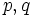# There exists an abelian group of prime power order that is lattice-isomorphic to a non-abelian group not of prime power order

## Statement

It is possible to have two groups$G_1, G_2$ such that:

• The lattice of subgroups of$G_1$ is isomorphic to the lattice of subgroups of$G_2$
•$G_1$ is a finite abelian group, finite nilpotent group, and group of prime power order
•$G_2$ is not a finite abelian group, finite nilpotent group, or group of prime power order.

## Proof

Choose primes$p,q$ such that$q$ divides$p - 1$. Choose the following:

•$G_1$ is the elementary abelian group of order$p^2$. It is clearly finite abelian, finite nilpotent, and of prime power order..
•$G_2$ is the external semidirect product of the cyclic group of order$p$ by the cyclic subgroup of order$q$ in its automorphism group. It is clearly non-abelian and not of prime power order (its order is$pq$).

Both$G_1$ and$G_2$ have a lattice of size$p+3$, including the trivial subgroup, whole group, and$p + 1$ intermediate, mutually incomparable subgroups. These lattices are clearly isomorphic.

The smallest example is$p = 3, q = 2$, giving$G_1$ as elementary abelian group:E9 and$G_2$ as symmetric group:S3.